Back

Back

Back

Back

## Tools & Support

• Public Relations
Precautions and Zero Offset Compensation Methods when using ADC of 8-bit G1 Series
• Written by manager
• Written date 2022-02-25 00:00:00
• Inquire 4166
Precautions and Zero Offset Compensation Methods
when using ADC of 8-bit G1 SeriesABOV’s 8-bit G1 series is a general purpose MCU that includes a 12-bit resolution Analog to Digital Converter (ADC). It can be used for controlling a secondary battery charger.

When using a charger, an A-D converter in a low voltage band generally requires high precision. Therefore, you need to be familiar with precautions and Zero Offset compensation methods before using ADC.

First, let’s explain how to use ADC and what to remember.

- The 12-bit ADC on each MCU of 8-bit G1 series has the same structure, but the number of input channels, triggers, and the support for external voltage reference can be different. Therefore, you need to check the block diagram of each MCU.

[Figure 1] shows the block diagram of the ADC in A96G174.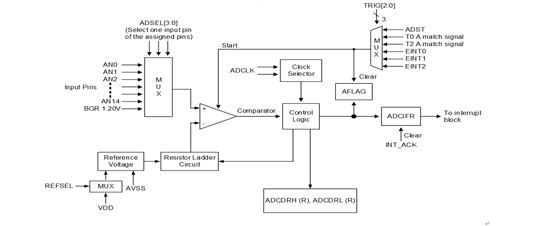[Figure 1] ADC Block Diagram (Example: A96G174)

[A-D conversion speed]

- The ADC of 8-bit G1 series is a Successive Approximation Register (SAR) ADC that has no Sample & Hold characteristics, therefore it can be used with the A-D conversion speed as fast as possible.

- A clock for the A-D conversion is allowed up to 8MHz. The A-D conversion process for one bit consists of four steps (4 clock edges) and requires 12 additional clocks.

- For example, assuming that the System Operating Clock (fx) is 16MHz, the maximum A-D conversion clock 8MHz can be used by dividing fx by 2. The calculation is as follows: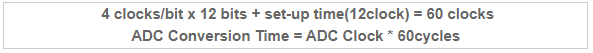The A-D conversion time is recommended to be greater than ‘(1/8MHz) x 60 clocks = 7.5us’ and as fast as possible.

[Reference voltage selection]
- You can use the VDD of the MCU as a reference voltage for A-D conversion (internal reference), or you can directly apply a reference voltage to the AVREF pin (external reference).

When the AVREF pin is used for the reference voltage of A-D conversion, the AVREF pin cannot be used for other purposes, but is less affected by noise than when the VDD is used as the reference voltage. Therefore, you can obtain more precision results, and specially for very small A-D input signals, lowering the AVREF voltage can achieve higher resolution.

The voltage applicable to the AVREF pin must be set to a level that is equal to or lower than the VDD, and at least 1.8V or higher. If the AVREF voltage is higher than the VDD, an unstable state occurs in which power is supplied from the AVREF pin to the VDD through the Pin Protection Diode. When using the AVREF pin for the A-D conversion, a correct DC voltage must be applied using capacitors.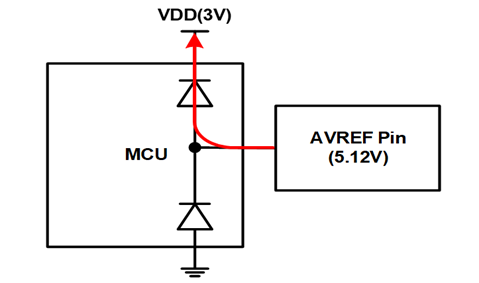[Figure 2] A Circuit Diagram where AVREF Voltage Is Higher than VDD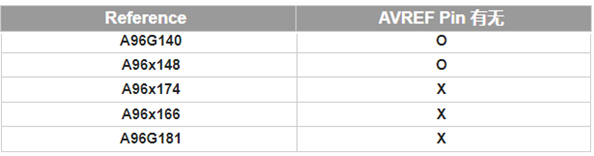[Table 1] Presence of AVREF Pin

[A-D input signal selection]

- The ideal input signal for A-D conversion is a complete DC signal without noise. To this end, it is important to input a band limited signal, and noise coming in through the input signal is limited using RC filters, in general.

If resistance value makes an effect to the input level, you can use only simple capacitors in some cases. The input signal for A-D conversion can be set according to the assigned pin. Usually, the last input signal is connected to the internal constant voltage power source (1.2V) without assigning to A-D input signal pin.

[Linearity characteristics]

- Differential Non-linearity (DNL) characteristic includes a phenomenon that a value stands out abnormally at a specific location. Integral Non-linearity (INL) characteristic is a factor that causes a gap from the ideal slope due to the accumulated errors, and can be described as the difference between the maximum value (0xFFF) and an input signal approaching the reference voltage.

[Zero Input Offset compensation]

- The high precision A-D conversion has a Zero Offset characteristic that causes critical errors when measuring the micro-voltage.

For example, assuming that you measure the end-of-charge current, based on the power supply voltage of 2.8V, the A-D conversion result of 5mV is theoretically a small value of 0x007 and the ADC Zero Input Offset management is an important item.

If Zero Offset value is -6, the A-D conversion result of 5mV is 0x001 which is not a valid value. In particular, the minus Offset values causes the A-D converter to read all low level signals of mV units as 0x000, making it impossible to measure. Therefore, to improve the precision, manufacturers should provide users with information regarding the Input Offset characteristics and the users should correct the Offset value of the A-D conversion result.

An Offset value that can identify the minimum input signal must be determined to define the effective limit of minus Offset value.

﻿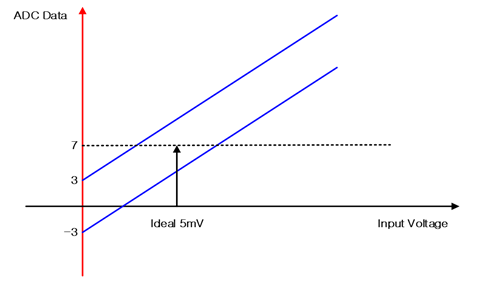[Figure 3] ADC Zero Offset Characteristics (Example: A96G174)

[Table 2] shows the memory location where each MCU stores the Input Offset value of the A-D converter. Before starting user program, read the Offset value of the MCU. And after A-D conversion, obtain a correct A-D conversion value by subtracting the Offset value from the read value of A-D conversion.

The Zero Offset Compensation is applied only when a low input voltage of 100mV or less is accurately measured. The reference voltage of the Zero Offset Compensation is the Internal Reference (VDD).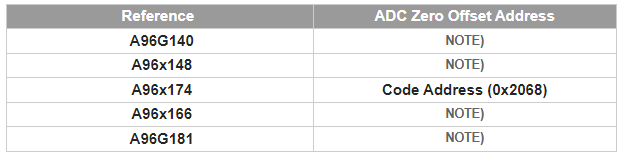NOTE) This device is scheduled to support this feature later.
[Table 2] ADC Zero Offset Storage Location

So far, we have explained precautions and Zero Offset Compensation methods when using ADC. Now we will introduce how to apply the Zero Offset using software and describe the ADC conversion algorithms.

- For the complete A-D conversion, you need to store the ADC Zero Offset value that is unique for each MCU. You can obtain a correct A-D conversion value by reading the ADC Zero Offset value at the location shown in Table 2 and subtracting it from the result value of the A-D conversion.

The ADC Zero Offset value is written to the memory location specified in Table 2, during the test process. The data type is ‘signed char’ and the measurement reference voltage is the Internal Reference (VDD).

[A-D Conversion algorithm]

- If noise is severe or the input signal fluctuation is large, you can use the mean filter algorithm or average filter algorithm to obtain average values. As the number of A-D conversions increases, more RAM (Buffer where the result value of A-D conversion is stored) is used but more accurate values can be obtained.

In this document, the result value of the A-D conversion will be the final value that is corrected by following the steps below:

1.     Read the initial result values of the A-D conversion, six times.

2.     Calculate the average of the remaining four values after excluding the largest and smallest of the six initial results of the A-D conversion.

3.     Subtract the unique ADC Zero Offset value stored during manufacture from the average value.

4.     Take the final value as the A-D conversion output value.

[Example code – Using ADC Zero Offset]

- The 8-bit G1 series basically has a similar A-D conversion process for each MCU. Examples below show that A96G174 applies the ADC Zero Offset value to its A-D conversion process. Since each MCU has a different ADC Zero Offset Address, you need to refer to [Table 2].

 #define CODEROM    ((char volatile code *) 0)#define OFFSET_ADDRESS (0x2068)                          // refer to offset address of each mcuchar adc_offset = 0;                                     // signed charvoid Get_ADC_Offset(void){          unsigned char IE_temp;          IE_temp = IE;                                   // Backup IE          IE = 0;                                          // Disable Global Interrupt          FESR |= 0x20;                                   // Remap Enable          adc_offset = CODEROM[OFFSET_ADDRESS];           // Read ADC Zero Offset Value          FESR &= ~(0x20);                                // Remap Disable          IE = IE_temp;                                   // Restore IE

▶ Setting 12-bit A-D Converter

 void main( void ){          ⁞          /* Initialize ADC */          ADCCRL = 0x00      // Initial                  | (1<<7)    // 1 : Enable ADC Module                  | (0<<6)    // 0 : A/D Conversation No effect                  | (0<<5)    // 0 : Internal Reference(VDD)                  | (0<<0)    // 0 : A/D Converter Input Select(AN0)                  ;                   ADCCRH = 0x00      // Initial                  | (0<<6)    // 0 : External Input Source Select(for use ANx)                  | (0<<3)    // 0 : ADST(ADC Conversion Start from User S/W)                  | (1<<2)    // 0 : LSB align                  | (3<<0)    // 3 : A/D Converter Clock Selection                                      (ADC clock should use below 8MHz)                  ;          ⁞          while(1)          {                   ⁞                   ADC_Mean_Average();                   ⁞          }}

▶  Mean & Average Algorithm